# Function Router

All the C# code examples presented on this page can be found in the related demo function of MS Visual Studio project FinaquantProtosStarter which can be downloaded at the product page of the free .NET library finaquant® protos.
As explained in the related article, subtable transformation is a simple way of applying the same table function on every subtable of an input table.

Function Router1 extends this concept further; with the table functions of the .net library finaquant® protos like `FunctionRouterA()` it is possible to apply selected table functions on selected subtables of an input table, as the examples below will illustrate.The entity attributes (all the text and numeric attributes of an input table excluding subtable fields) determine which table function is to be applied on which subtable (sub-data of an entity).

Following scenarios may give you an idea about the use of function routers in practice:

1. You want to calculate expected population of some cities in ten years from now depending on historical data like birth rate, population, migration rate and so on. You have an input table containing historical data for many cities, and depending on some attributes of the cities (entity attributes) like size (small, medium, big), altitude (low, high), average income category etc., you need to apply different formulas (table functions) for different cities to obtain the estimated population for each city in a result table.
2. Scenario analysis: For the same scenario above, you may want to calculate the expected population for each city under different discrete scenarios like optimistic, realistic and pessimistic, and you may need to apply totally different formulas (or functions), each with its own set of additional parameters, to compute the expected populations for each scenario (see 2. example below).
3. You want to calculate a performance measure for some financial assets, and for each asset group (entity attribute) you need to apply a group-specific performance function (table function). You have an input table containing data for multiple assets belonging to several asset groups, and you want to obtain the performance measure for each asset in a result table.
4. For the same scenario above, you might have written your performance functions with another library for matrix computations like ILNumerics, R or matlab. You want to calculate the performance of each asset by feeding its subtable to its group-specific matrix function, provided that all the subtable fields are numbers; that is, either numeric attributes or key figures in the finaquant data structure (see 4. example below).
5. You have some numbers like estimations, prices, costs etc. related with some entities like city, product, or invoice in a table. You want to check and validate these numbers, and generate warnings if something is wrong. For each entity-group you may need to apply a different set of validation functions (table or matrix functions). In that sense, a function router can also work like a rules engine! With the parameters of a function router you can set dynamically which validation functions are to be applied on which entities (see 5. example below).

## Result table of a function router

You may see below the structure of a result table that is produced by a function router: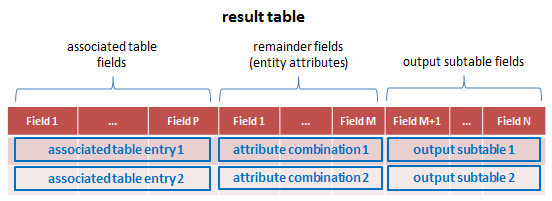The resultant (output) subtables are combined with:

1. Corresponding entity attribute combination of the input subtable which was directed to a certain table function to generate the output subtable.
2. The entry (row) of the associated table corresponding to the particular table function applied on the input subtable.

The associated table as parameter to a function router can be left empty if you don’t want to add extra fields to the result table.

For example, assume you want to calculate the expected population for some cities in ten years from now. Your input table has following fields:

1. Entity attributes: city_category (small, medium, large), city_name
2. Subtable fields: year, population (i.e. historical population)
3. Table functions: For each city category you need to apply different table functions on the subtables: TF_small() and TF_large(), where TF_large() is also applied for medium-sized cities.

In order to have the highest transparency about the applied estimation methods, you may want to add an additional field into your result table: estimation_method with possible values small city or large city. You can add this field into the result table by using the parameter associated table.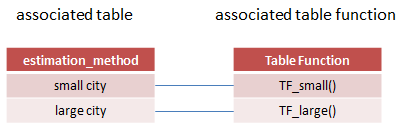The result table will then look like as follows: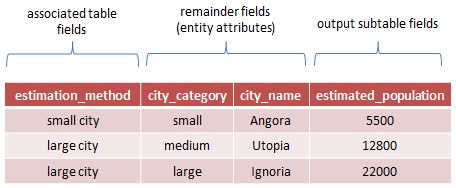## Anatomy of a table function as Function Router

```// Function router with condition table as filtering method. // Feed selected subtables of primary input table (BaseTbl) // that match i'th row of condition table to corresponding table function. public static MatrixTable FunctionRouterA(MatrixTable BaseTbl, TableFields SubTableFields, MatrixTable CondTbl, TransformTableFunc_OP[] MyTableFuncList, bool JokerMatchesAllvalues = false, string TextJoker = "ALL", int NumJoker = 0, bool FirstMatchOnly = false, params object[][] OtherParametersList)```

### Input parameters

1. BaseTbl: Input table with entity attributes and subtable fields.
2. SubTableFields: Subtable fields of BaseTbl. Each subtable with these fields are directed to a certain table function to generate output subtables.
3. CondTbl: Condition table for filtering BaseTbl in order to determine which subtables are fed to which table function. Only the entity attributes of BaseTbl are relevant for filtering. First row of the condition table is associated with the first table function, second row with the second table function, and so on. All the subtables of entity attribute combinations that match to the i’th row of condition table are directed to i’th table function.
4. MyTableFuncList: Array of table functions (as delegate function), each corresponding to a row of condition table.
5. JokerMatchesAllvalues: If true, joker (match-all) values match all field values; for conditional filtering.
6. TextJoker: Match-all value for text attributes; relevant only if JokerMatchesAllvalues is true.
7. NumJoker: Match-all value for numeric attributes; relevant only if JokerMatchesAllvalues is true.
8. FirstMatchOnly: If true, a row of BaseTable can be matched with only a single row of condition table with from-top-to-down as priority order. Setting FirstMatchOnly to false is useful for cases like scenario analysis.
9. OtherParametersList: Set of parameters of any type for each table function. This parameter can also be left empty which means there is no additional parameter for any table function. If there is only one set of parameters in the array, this parameter set is shared by all table functions.

## Examples

I will use the same example case here as presented in the previous article subtable transformation with some variations:

Computing expected average energy consumption for household heating next year in May, in several cities based on historical data like population and temperature.

Following test table with historical monthly average temperatures and population will be used for all the examples below as the primary input.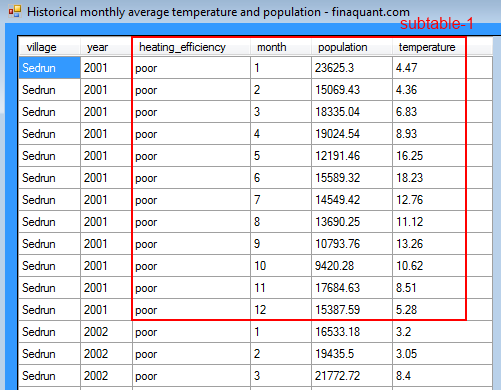This input table contains average monthly temperatures for the years from 2001 to 2012. The task is calculating expected energy consumption for each village and year in mega-joules2. You may download this input table as an excel file for a closer check:

### Example 1: Apply the same user-defined table function on all subtables

In this example, the same user-defined function is applied on every subtable, just like a subtable transformer. That is, the same function is applied for every village-year pair as entity attributes.

The user defined table function `ExpectedEnergyConsumption()` encapsulates following estimation formula:

`ExpectedEnergyConsumption (per month) = Population ÷ (50 + Temperature) x EfficiencyMultiplier`

As we are interested in yearly energy consumption estimates, per month estimations are summed up in the user-defined function to obtain the yearly estimate.

```/// User-defined table function for calculating expected yearly energy consumption (Mega-Joules) for heating; method A /// For each month: (hypothetical formula) /// Expected energy consumption = population / (50 + temperature) x EfficiencyMultiplier public static MatrixTable ExpectedEnergyConsumption(MatrixTable TemperatureSubTbl, params Object[] Parameters) { // PARAMETER CHECKS // table function for demonstration purposes, no elaborate parameter checks; // assumes parameters are entered correctly. MatrixTable EfficiencyMultTbl = (MatrixTable)Parameters;   // combine input tables to have all parameters in a single table MatrixTable CombinedTbl = MatrixTable.CombineTablesFirstMatch(TemperatureSubTbl, EfficiencyMultTbl); // declare variables double Population, Temperature, Efficiency_Multiplier, Energy_Consumption = 0.0; TableRow trow;   // sum over months for (int i = 0; i < CombinedTbl.RowCount; i++) { trow = CombinedTbl.GetTableRow(i);   // get parameters Population = TableRow.GetKeyFigureValue(trow, "population"); Temperature = TableRow.GetKeyFigureValue(trow, "temperature"); Efficiency_Multiplier = TableRow.GetKeyFigureValue(trow, "efficiency_multiplier");   // calculate energy consumption Energy_Consumption += Population / (50 + Temperature) * Efficiency_Multiplier; } // Return table with single key figure "energy_consumption" var EnergyConsumptionFields = TableFields.CreateEmptyTableFields(CombinedTbl.metaData); TableFields.AddNewField(EnergyConsumptionFields, "energy_consumption"); // return output table return MatrixTable.CreateTableWithElements_A(EnergyConsumptionFields, Energy_Consumption); }```

Now apply the user-defined table function above on all subtables of the primary input table. Note that all the example C# code presented here including the user-defined functions (and more) can be found in the Visual Studio project FinaquantProtosStarter (in releases 1.02 and higher).

```// define subtable fields var SubTblFields = TableFields.CreateEmptyTableFields(md); TableFields.AddNewField(SubTblFields, "heating_efficiency"); TableFields.AddNewField(SubTblFields, "month"); TableFields.AddNewField(SubTblFields, "population"); TableFields.AddNewField(SubTblFields, "temperature");   // create condition table var CondTblFields = TableFields.CreateEmptyTableFields(md); TableFields.AddNewField(CondTblFields, "village"); TableFields.AddNewField(CondTblFields, "year");   var CondTbl = MatrixTable.CreateTableWithElements_A(CondTblFields, "ALL" , 0 // match all vilages and years ); // view condition table MatrixTable.View_MatrixTable(CondTbl, "CASE 1: Condition table");``````// create associated table var AssocTblFields = TableFields.CreateEmptyTableFields(md); TableFields.AddNewField(AssocTblFields, "estimation_method");   var AssocTbl = MatrixTable.CreateTableWithElements_A(AssocTblFields, "Method A" // field value(s) to be added to corresponding subtable outputs ); // array for delegate functions var MyTableFuncList = new TransformTableFunc_OP; MyTableFuncList = UserFunctions.ExpectedEnergyConsumption; // array for other parameters var OtherParametersList = new object[]; OtherParametersList = new object[] { EffMultiplierTbl }; // call Function Router MatrixTable ResultTbl = MatrixTable.FunctionRouterA(TemperatureTable, SubTblFields, CondTbl, MyTableFuncList, AssocTbl, JokerMatchesAllvalues: true, TextJoker: "ALL", NumJoker: 0, FirstMatchOnly: true, OtherParametersList: OtherParametersList); // round all key figures to 2 digits after decimal point ResultTbl = MatrixTable.TransformKeyFigures(ResultTbl, x => Math.Round(x, 2), InputKeyFig: null, OutputKeyFig: null); // view result with ordered fields OrderedFields = TextVector.CreateVectorWithElements("village", "year", "estimation_method", "energy_consumption"); MatrixTable.View_MatrixTable(ResultTbl, "CASE 1: Result table", OrderedFields);```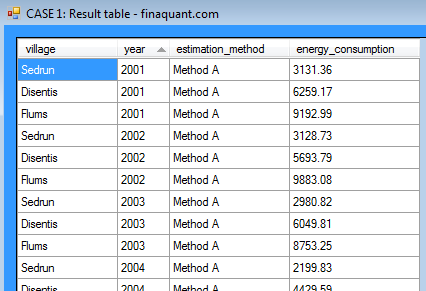As you may see in the cross-section of the result table above, the same estimation function A is applied on all village-year pairs.

### Example 2: Apply different user-defined table functions on different subtables

In this example, there are two estimation methods, A and B. Accordingly, there are two slightly different user-defined table functions to be applied on different subtables. Two parameters of the function router determine, which method is applied on which subtables: Condition table (CondTbl) and function array (MyTableFuncList).

```// Condition table: // Apply old estimation method (B) for years 2001 and 2002; new method A from 2003 on // Apply old estimation method (B) for Sedrun also for years 2003 and 2004 CondTbl = MatrixTable.CreateTableWithElements_A(CondTblFields, "ALL", 2001, "ALL", 2002, "Sedrun", 2003, "Sedrun", 2004, "ALL", 0 ); // view condition table MatrixTable.View_MatrixTable(CondTbl, "CASE 2: Condition table");```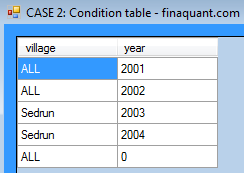```// array of user-defined delegate functions; assign a function for each row of condition table MyTableFuncList = new TransformTableFunc_OP; MyTableFuncList = UserFunctions.ExpectedEnergyConsumption_B; // method B MyTableFuncList = UserFunctions.ExpectedEnergyConsumption_B; MyTableFuncList = UserFunctions.ExpectedEnergyConsumption_B; MyTableFuncList = UserFunctions.ExpectedEnergyConsumption_B; MyTableFuncList = UserFunctions.ExpectedEnergyConsumption; // method A // array for other parameters; only last function requires an additional parameter OtherParametersList = new object[]; OtherParametersList = new object[] { EffMultiplierTbl }; // call Function Router ResultTbl = MatrixTable.FunctionRouterA(TemperatureTable, SubTblFields, CondTbl, MyTableFuncList, AssocTbl, JokerMatchesAllvalues: true, TextJoker: "ALL", NumJoker: 0, FirstMatchOnly: true, OtherParametersList: OtherParametersList); // round all key figures to 2 digits after decimal point ResultTbl = MatrixTable.TransformKeyFigures(ResultTbl, x => Math.Round(x, 2), InputKeyFig: null, OutputKeyFig: null); // view result with ordered fields OrderedFields = TextVector.CreateVectorWithElements("village", "year", "estimation_method", "energy_consumption"); MatrixTable.View_MatrixTable(ResultTbl, "CASE 2: Result table", OrderedFields);```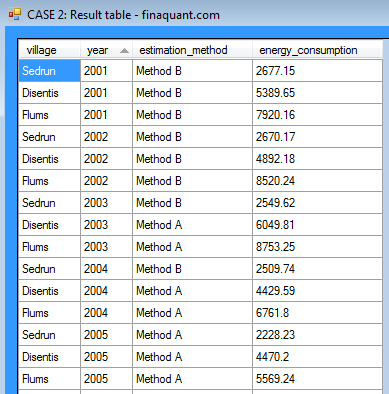As you may see in the result table above, the old estimation method B was applied all other villages until 2002; only for Sedrun until 2004.

### Example 3: Apply both estimation methods on all village-year pairs

In this example, the user wants to see the estimation results for both methods A and B, for all village-year pairs (entity attributes) by setting the critical parameter FirstMatchOnly to false. FirstMatchOnly = false means, a subtable of input table can be matched with multiple rows of a condition table.

This example also illustrates, how a function router can be used for scenario analysis. You ask a “what happens if” question, and want to see the outcomes for all possible discrete cases. Each case may require a different logic, and a different function with its own set of additional parameters to compute the expected outcomes.

```// Condition table: CondTbl = MatrixTable.CreateTableWithElements_A(CondTblFields, "ALL", 0, "ALL", 0 ); // create associated table AssocTbl = MatrixTable.CreateTableWithElements_A(AssocTblFields, "Method A", "Method B" ); // array for delegate functions; assign function for each row of condition table MyTableFuncList = new TransformTableFunc_OP; MyTableFuncList = UserFunctions.ExpectedEnergyConsumption; // method A MyTableFuncList = UserFunctions.ExpectedEnergyConsumption_B; // method B // array for other parameters; only first function (A) requires an additional parameter OtherParametersList = new object[]; OtherParametersList = new object[] { EffMultiplierTbl }; // call Function Router, NOTE: FirstMatchOnly = false ResultTbl = MatrixTable.FunctionRouterA(TemperatureTable, SubTblFields, CondTbl, MyTableFuncList, AssocTbl, JokerMatchesAllvalues: true, TextJoker: "ALL", NumJoker: 0, FirstMatchOnly: false, OtherParametersList: OtherParametersList); // round all key figures to 2 digits after decimal point ResultTbl = MatrixTable.TransformKeyFigures(ResultTbl, x => Math.Round(x, 2), InputKeyFig: null, OutputKeyFig: null); // view result with ordered fields OrderedFields = TextVector.CreateVectorWithElements("village", "year", "estimation_method", "energy_consumption"); MatrixTable.View_MatrixTable(ResultTbl, "CASE 3: Result table", OrderedFields);```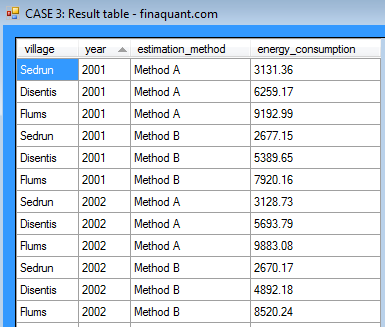As seen in the result table above, both estimation methods A and B are applied on all subtables of village-year pairs.

### Example 4: Matrix Function Router with Condition Matrix Table as filtering method

As explained in a related article, condition table is only one of the filtering methods available in finaquant libraries. There are two other filtering methods, namely condition cell array and condition matrix table.

This example illustrates two major points:

1. Selecting subtables with the filtering method condition matrix table
2. Using user-defined matrix functions as table transformer, provided that all subtable fields are numbers; that is, either numeric attributes or key figures.

Note that the user-defined matrix function can be used as just an envelope for a function written with the methods of another library. This construct makes the integration of a function router with the matrix functions of other libraries possible and feasible. Look for our upcoming articles at finaquant.com about this integration issue.

```// Replace text attribute heating_efficiency with key figure efficiency_multiplier in input table // so that all subtable fields are numbers as required by matrix function router   // combine tables in order to add the key figure efficiency_multiplier into table TemperatureTable = MatrixTable.CombineTablesFirstMatch(TemperatureTable, EffMultiplierTbl); // exclude text attribute heating_efficiency from table TemperatureTable = MatrixTable.ExcludeColumns(TemperatureTable, TextVector.CreateVectorWithElements("heating_efficiency")); // view temperature table with ordered fields OrderedFields = TextVector.CreateVectorWithElements("village", "year", "month", "efficiency_multiplier", "population", "temperature"); MatrixTable.View_MatrixTable(TemperatureTable, "Input table with numeric subtable fields", OrderedFields);```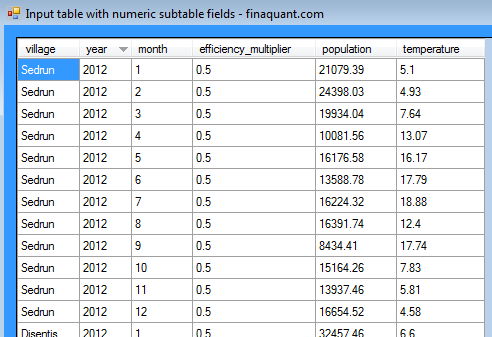```// add new fields to MetaData with proper string pattern for a condition matrix MetaData.AddNewField(md, "village_cell", FieldType.TextAttribute); MetaData.AddNewField(md, "year_cell", FieldType.TextAttribute); // define condition matrix table fields var CondMatrixTblFields = TableFields.CreateEmptyTableFields(md); TableFields.AddNewField(CondMatrixTblFields, "village_cell"); TableFields.AddNewField(CondMatrixTblFields, "year_cell");   // create condition matrix table // apply old estimation method B for years from 2001 to 2005 for all villages; // ... and for years 2006-2008 for Sedrun only // apply new estimation method A for years from 2006 to 2050 for all villages, // ... with the exception of Sedrun for years 2006-2008 MatrixTable CondMatrixTbl = MatrixTable.CreateTableWithElements_A(CondMatrixTblFields, "ALL", "[2001, 2005]", // to method B "Sedrun", "[2006, 2008]", // to method B "ALL", "[2006, 2050)" // to method A ); // view condition table MatrixTable.View_MatrixTable(CondMatrixTbl, "CASE 2: Condition matrix table");``````// create associated table AssocTbl = MatrixTable.CreateTableWithElements_A(AssocTblFields, "Method B", "Method B", "Method A" ); // array for delegate functions; assign function for each row of condition table MyMatrixFuncList = new TransformSubMatrixFunc_OP; MyMatrixFuncList = UserFunctions.ExpectedEnergyConsumptionMatrix_B; // method B MyMatrixFuncList = UserFunctions.ExpectedEnergyConsumptionMatrix_B; // method B MyMatrixFuncList = UserFunctions.ExpectedEnergyConsumptionMatrix; // method A // no additional parameter is required OtherParameters = null; // call matrix function router ResultTable = MatrixTable.MatrixFunctionRouterC(TemperatureTable, NumAttributesIn, KeyFiguresIn, NumAttributesOut, KeyFiguresOut, CondMatrixTbl, MyMatrixFuncList, AssocTbl, FirstMatchOnly: true, IgnoreHierarchy: true, ErrorIfConditionNotRelevant: false, OtherParametersList: OtherParameters); // round all key figures to 2 digits after decimal point ResultTable = MatrixTable.TransformKeyFigures(ResultTable, x => Math.Round(x, 2), InputKeyFig: null, OutputKeyFig: null); // view result with ordered fields OrderedFields = TextVector.CreateVectorWithElements("village", "year", "estimation_method", "energy_consumption"); MatrixTable.View_MatrixTable(ResultTable, "CASE 2: Result table", OrderedFields);```As seen in the result table above, the estimation method B is applied for the village Sedrun until 2008, whereas this method is applied for all other villages until 2005.

### Example 5: Function Router working like a rules engine for validations

In this example, the data (i.e. subtables) in the primary input table are not used to compute estimated energy consumptions; they are just used to calculate the lower and upper limits for estimations.

The estimations come from an external source, and the function router is used to validate these numbers by checking the lower and/or higher limit conditions.

Two user-defined table functions are used for validation of estimated energy consumptions per village and year:

1. `EnergyConsumption_CheckLowerLimit()`: Computes lower limit for a subtable, and returns “passed” or “not passed” as a value for the field validation_result
2. `EnergyConsumption_CheckUpperLimit()`: Computes higher limit for a subtable, and returns “passed” or “not passed” as a value for the field validation_result

The condition table (CondTbl) and the function array (MyTableFuncList) parameters of the function router determine, which validation functions are to be applied on which subtable for each village-year pair. In this way, one can dynamically define (or configure) the assignment of validation functions for each entity combination.

Note that you could have as many validation functions as you wish; not just two as demonstrated in this example.

```// add new fields to MetaData MetaData.AddNewField(md, "validation_result", FieldType.TextAttribute); MetaData.AddNewField(md, "validation_method", FieldType.TextAttribute);   // apply both lower and upper limit validations for Sedrun-2012 // apply only upper limit validation for Disentis-2012 // apply only lower limit validation for Flums-2012   // Condition table: CondTbl = MatrixTable.CreateTableWithElements_A(CondTblFields, "Sedrun", 2012, // apply lower limit "Sedrun", 2012, // apply upper limit "Disentis", 2012, "Flums", 2012 ); // array for delegate functions; assign function for each row of condition table MyTableFuncList = new TransformTableFunc_OP; MyTableFuncList = UserFunctions.EnergyConsumption_CheckLowerLimit; MyTableFuncList = UserFunctions.EnergyConsumption_CheckUpperLimit; MyTableFuncList = UserFunctions.EnergyConsumption_CheckUpperLimit; MyTableFuncList = UserFunctions.EnergyConsumption_CheckLowerLimit; // create associated table AssocTblFields = TableFields.CreateEmptyTableFields(md); TableFields.AddNewField(AssocTblFields, "validation_method");   AssocTbl = MatrixTable.CreateTableWithElements_A(AssocTblFields, "Lower Limit", "Upper Limit", "Upper Limit", "Lower Limit" ); // array for other parameters: Estimated Energy Consumtions OtherParametersList = new object[]; OtherParametersList = new object[] { 1700.0 }; // Sedrun-2012 OtherParametersList = new object[] { 1700.0 }; // Sedrun-2012 OtherParametersList = new object[] { 3200.0 }; // Disentis-2012 OtherParametersList = new object[] { 6500.0 }; // Flums-2012 // call function router ResultTbl = MatrixTable.FunctionRouterA(TemperatureTable, SubTblFields, CondTbl, MyTableFuncList, AssocTbl, JokerMatchesAllvalues: true, TextJoker: "ALL", NumJoker: 0, FirstMatchOnly: false, OtherParametersList: OtherParametersList); // view result with ordered fields OrderedFields = TextVector.CreateVectorWithElements("village", "year", "validation_method", "validation_result", "energy_consumption"); MatrixTable.View_MatrixTable(ResultTbl, "CASE 6: Result table", OrderedFields);```As seen in the result table above, both validation methods (Lower and Higher Limits) are applied on the village Sedrun, as it was configures by the condition table.
Written by: Tunç Ali Kütükçüoglu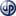Copyright secured by Digiprove © 2013 Tunc Ali Kütükcüoglu
extends this concept further;" data-image="http://software.tuncalik.com/wp-content/uploads/2013/01/FunctionRouter_Subtables.png" data-button="">1. The term “function router” is first used by the author of this article in this context and meaning. []
2. All the numbers and formulas used here for demonstration purposes are totally hypothetical without any relation to reality. []
3. The term "function router" is first used by the author of this article in this context and meaning. []
This entry was posted in Calculation engine and tagged , , . Bookmark the permalink.

### 2 Responses to Function Router

1.selmar says:

Function router can be a very useful function for those who spend hours and hours for converting some parts of tables into matrices on a case-by-case basis like myself. I often use R for matrix computations and I would like to see an example how function router can be integrated with R, as you mention in the article.

•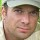tuncalik says:

Hello selmar, you can find an example in the related article: Table data as input to estimation functions in R – How can we apply estimation functions in R on selected parts (i.e. subtables) of an input table with historical data http://software.tuncalik.com/table-data-as-input-to-estimation-functions-in-r/3082